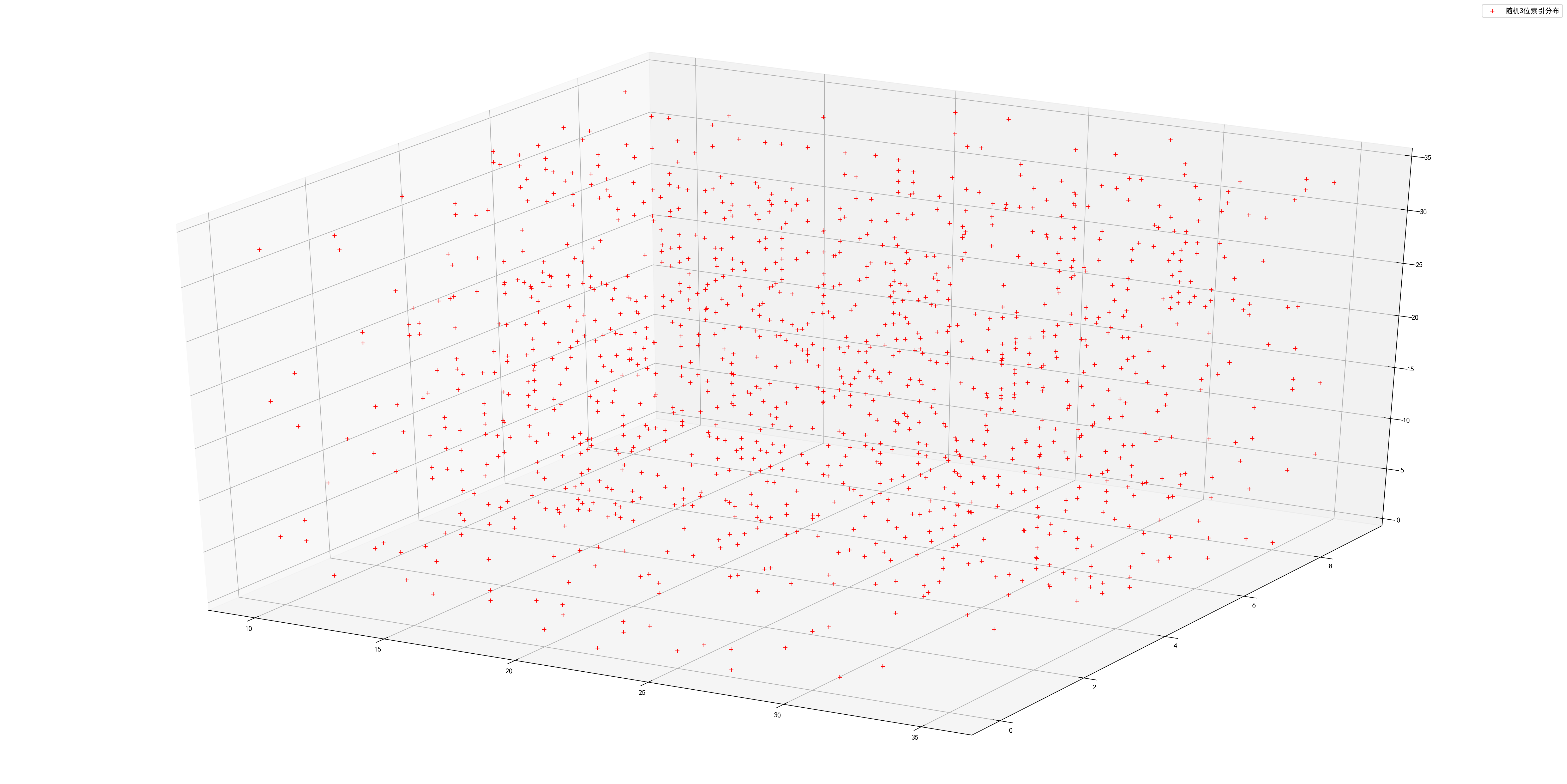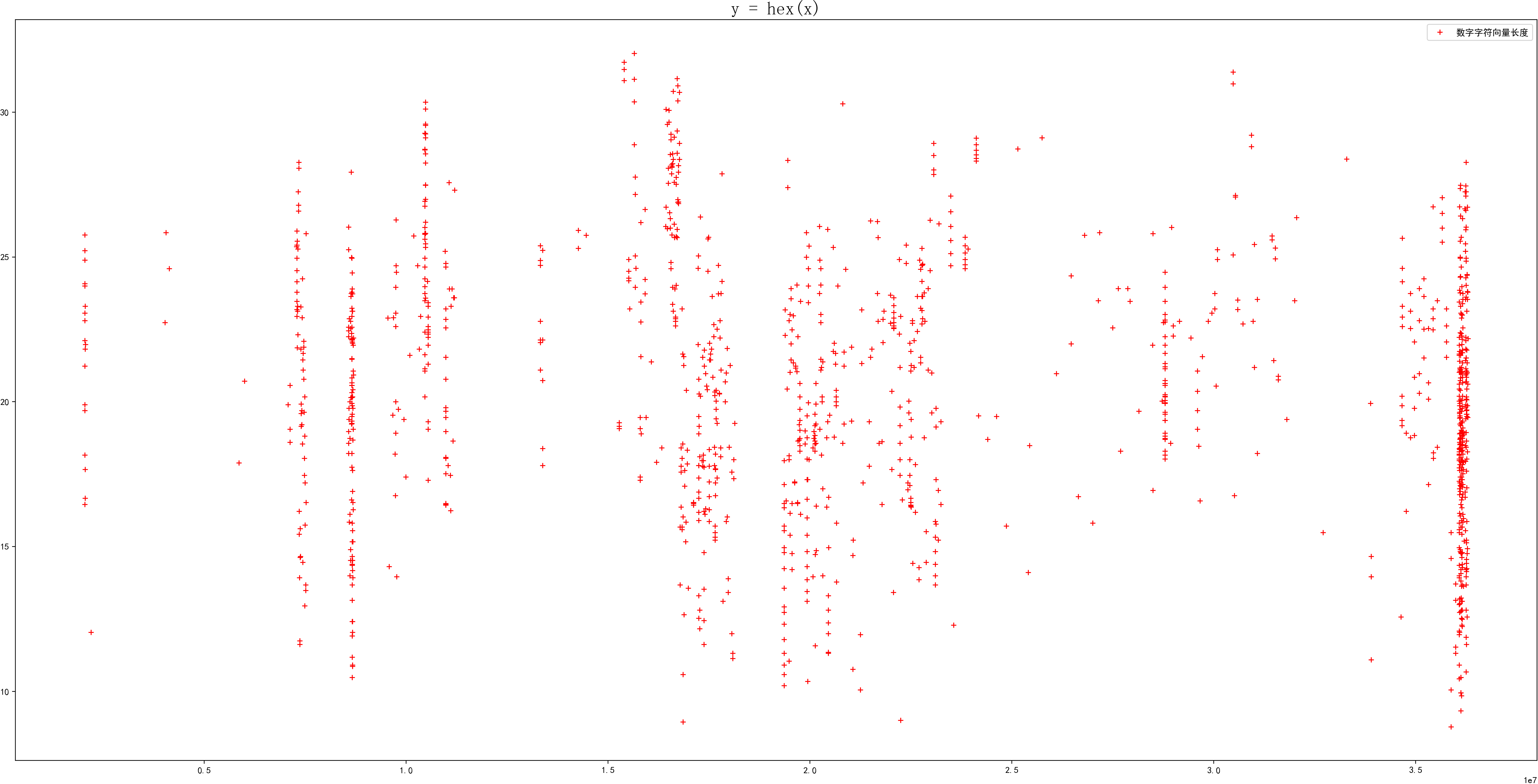# 某视频网站vid分析

``vlist = ['x0030wh28ov', 'p0024ht72c2', ..., 'x002483emfm', 'o0024m4oidi', 'n0024mvc1b8']``

## 字符频率

``````def cc(s):
ac = ['0', '1', '2', '3', '4', '5', '6', '7', '8', '9', 'a', 'b', 'c', 'd', 'e', 'f', 'g', 'h', 'i', 'j', 'k', 'l', 'm', 'n', 'o', 'p', 'q', 'r', 's', 't', 'u', 'v', 'w', 'x', 'y', 'z']
cres = [s.count(c) for c in ac]#统计字符出现次数
cresd = [x-y for x, y in zip(cres, cres[1:])]#相邻字符的差值
print(cres)
print(cresd)
print(sum(cresd))
string = ""
for vid in vlist:
string = string + vid[:1] + vid[5:]
cc(string)``````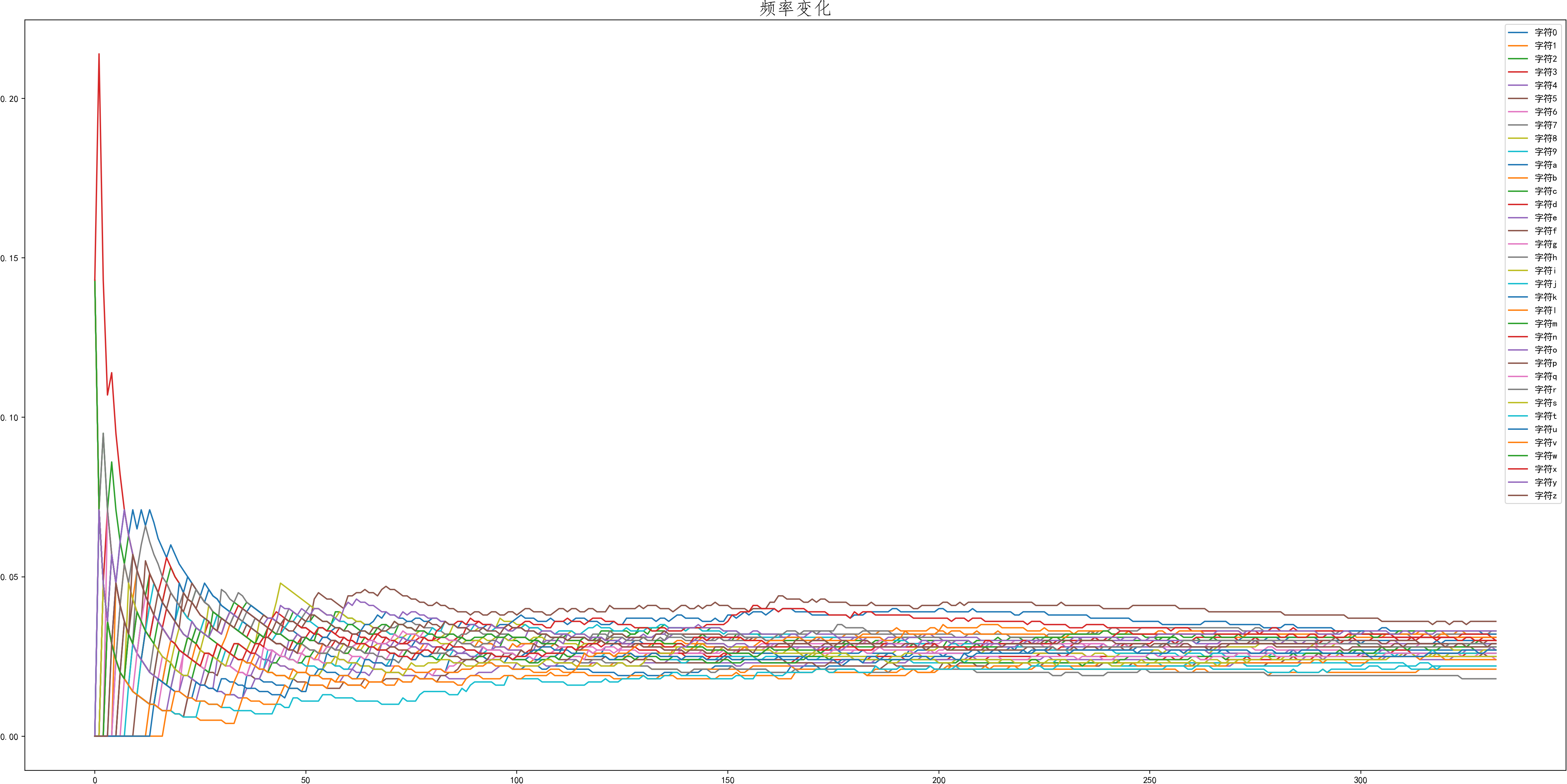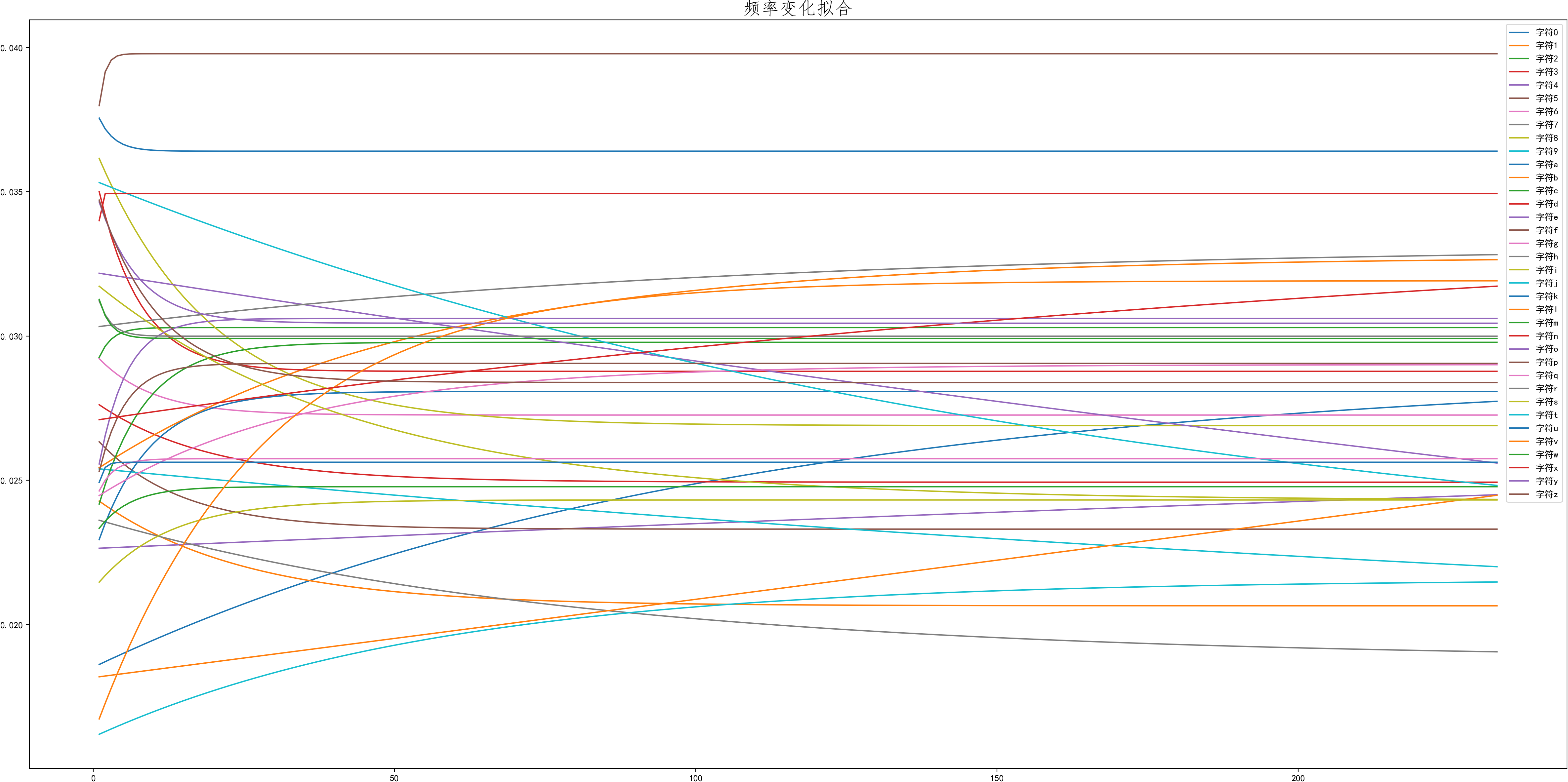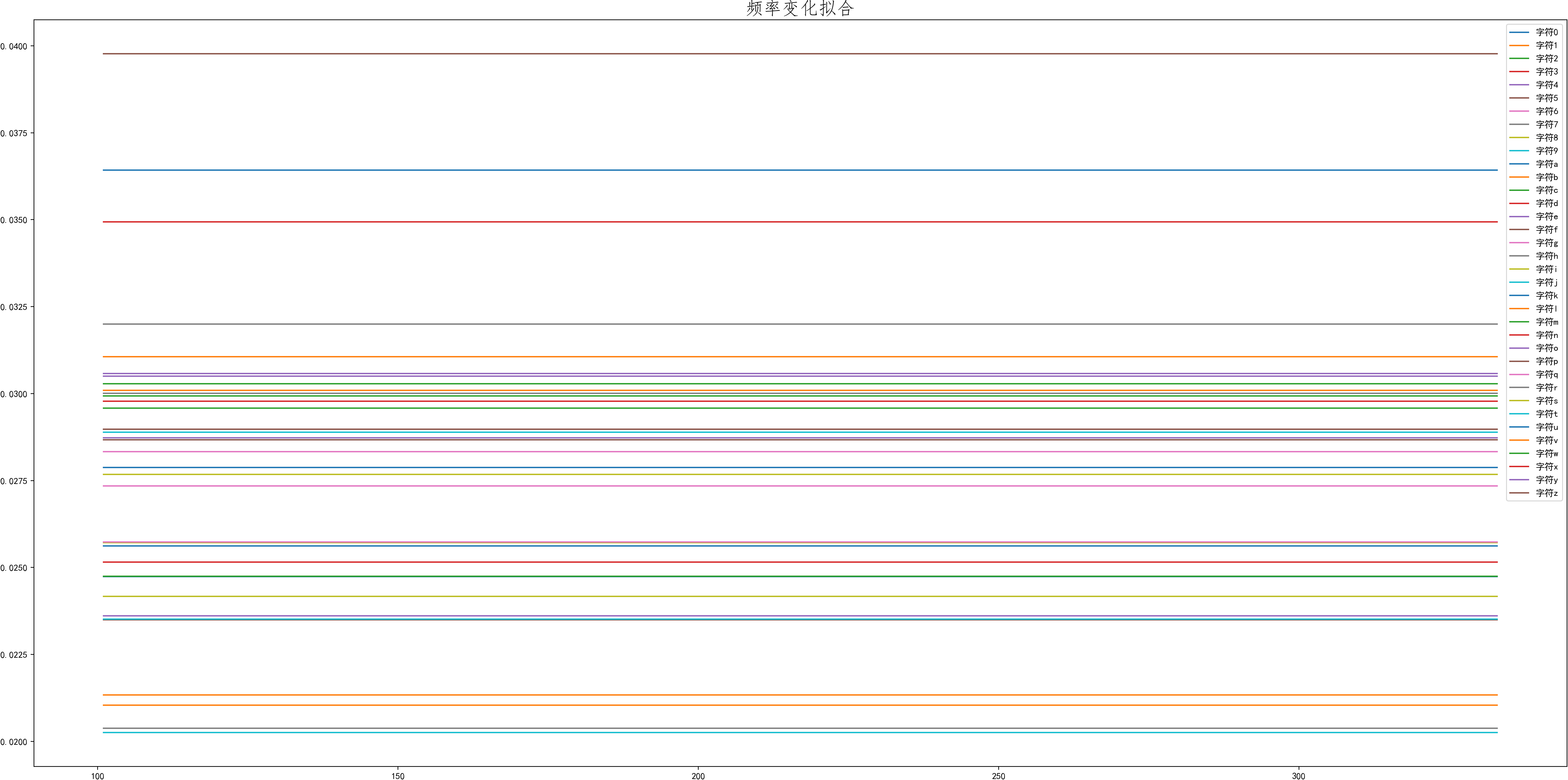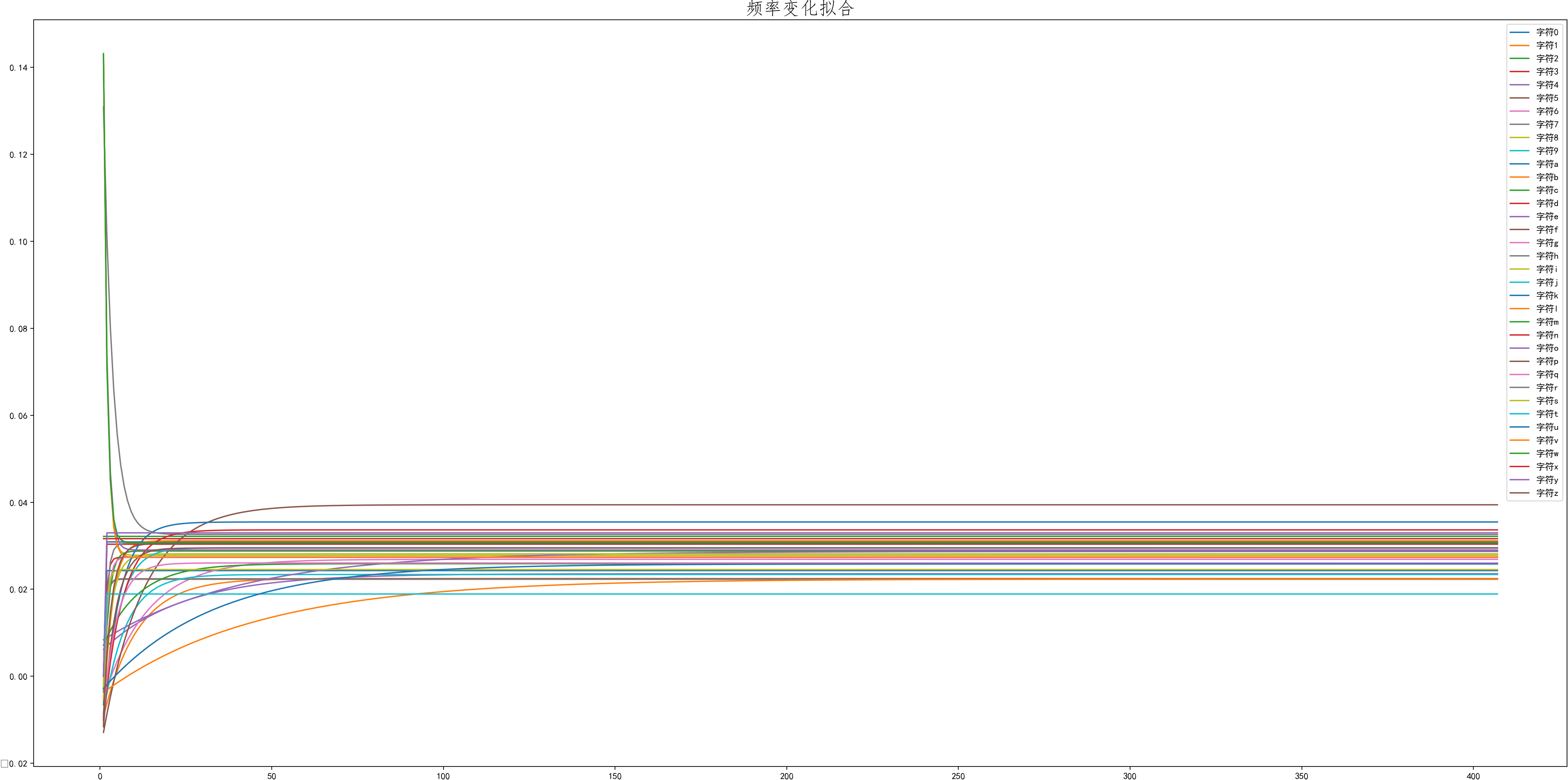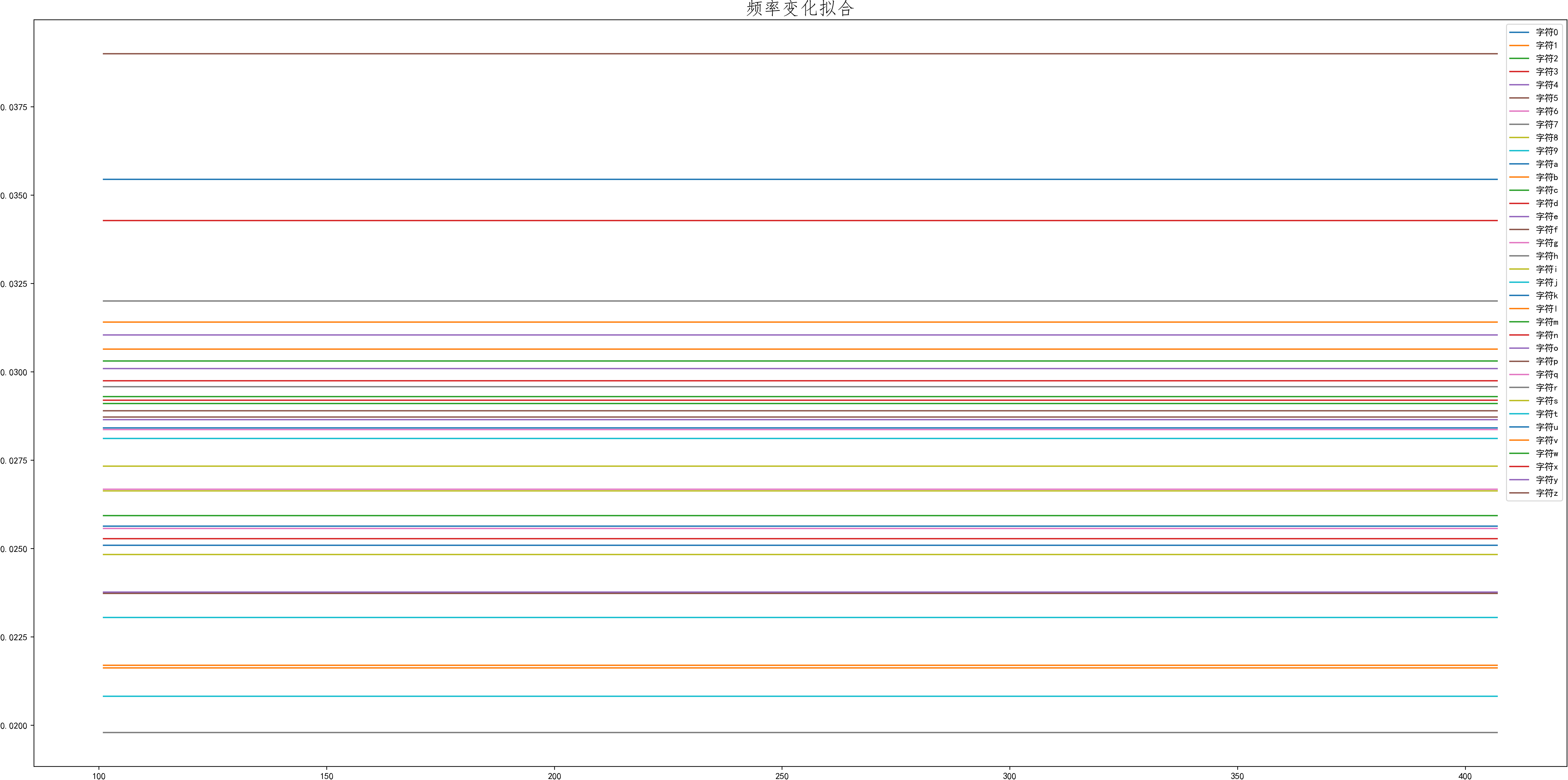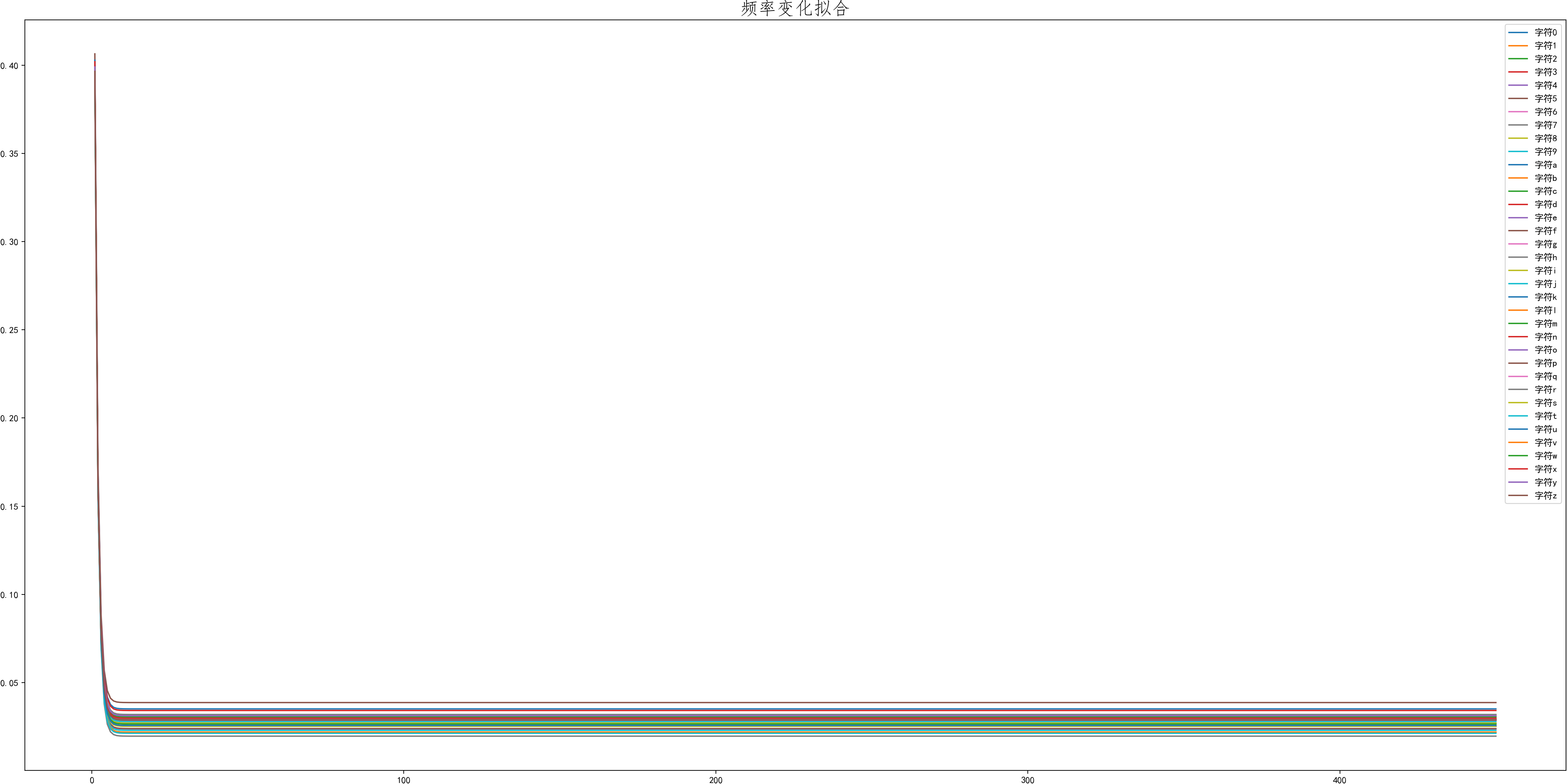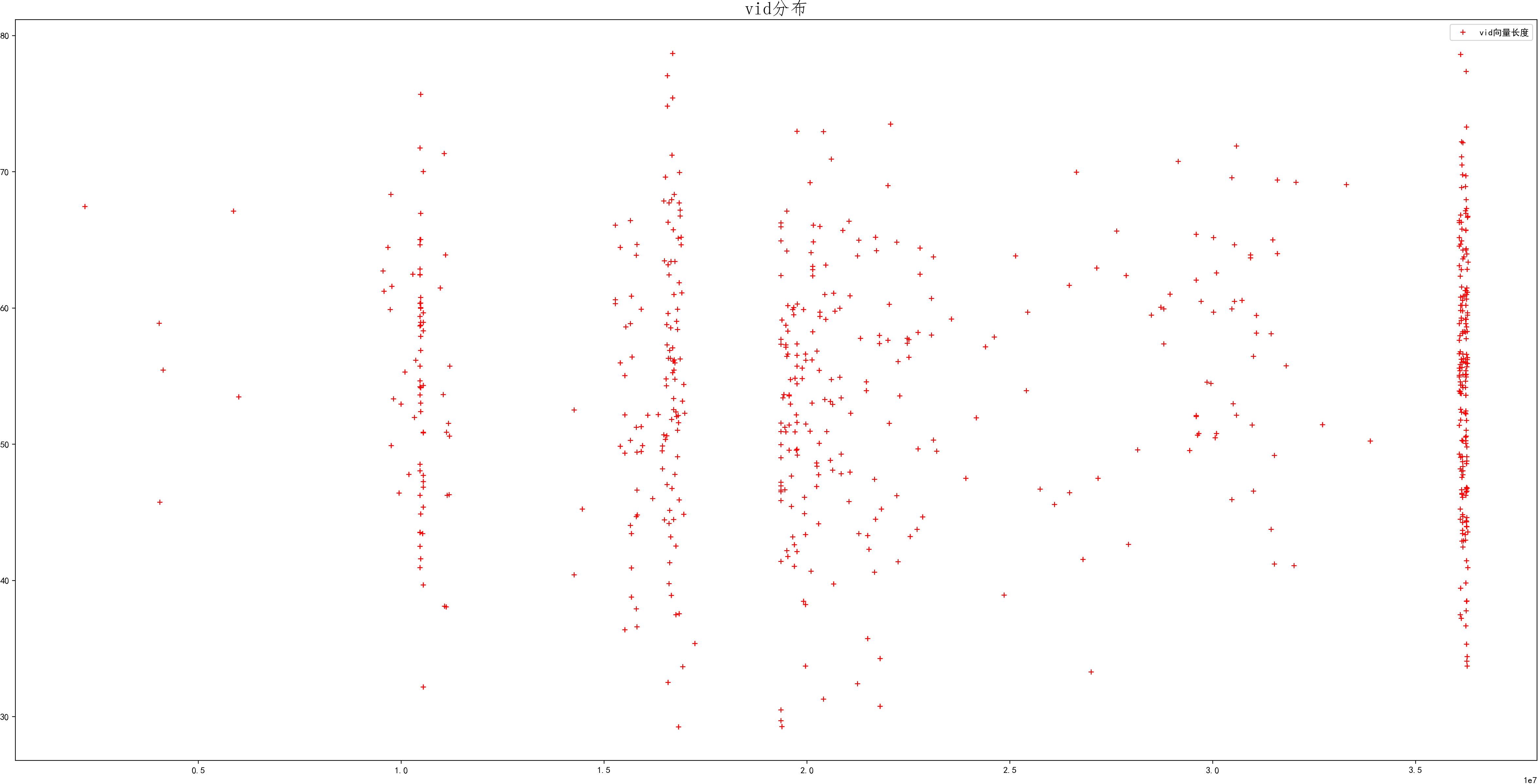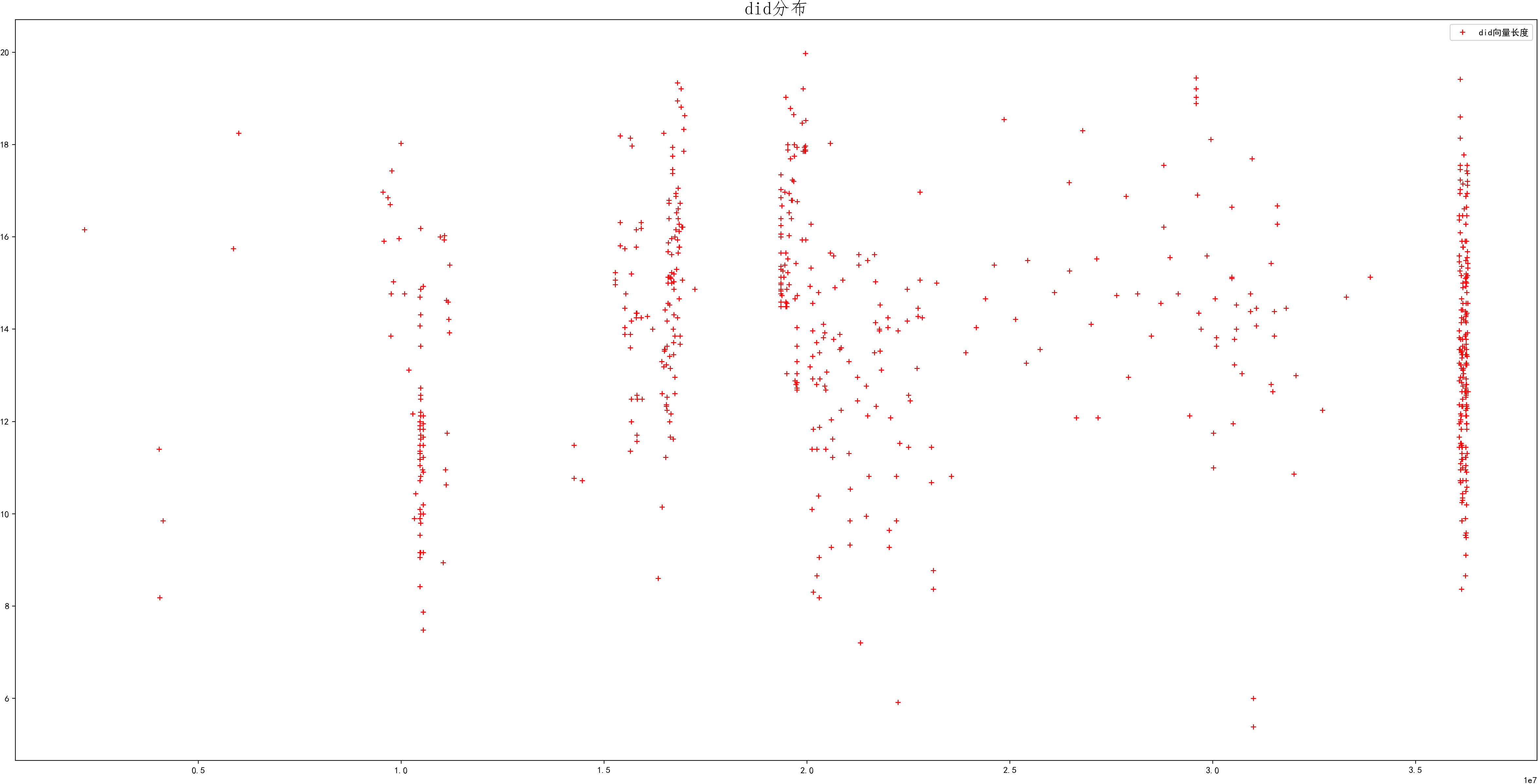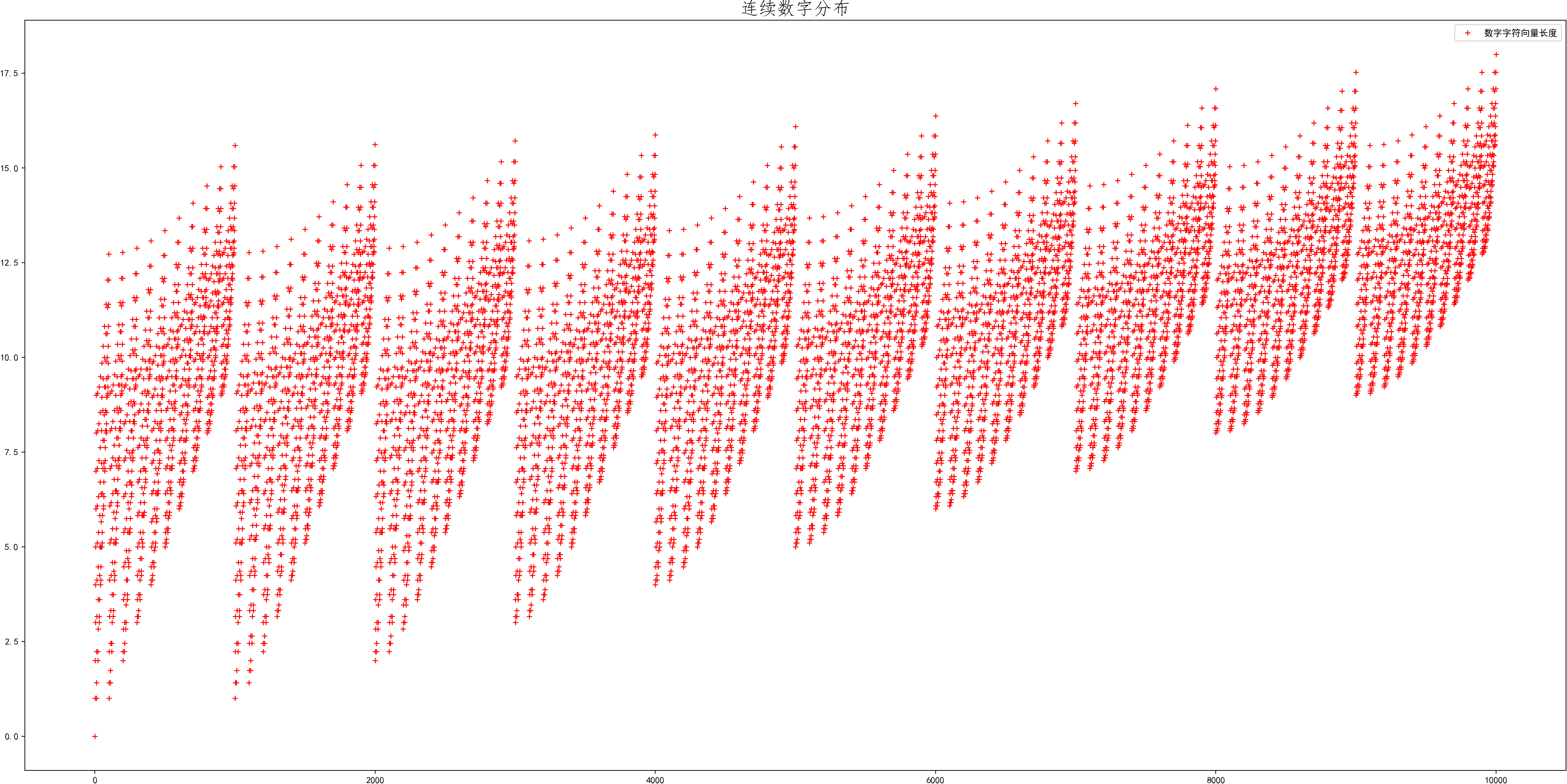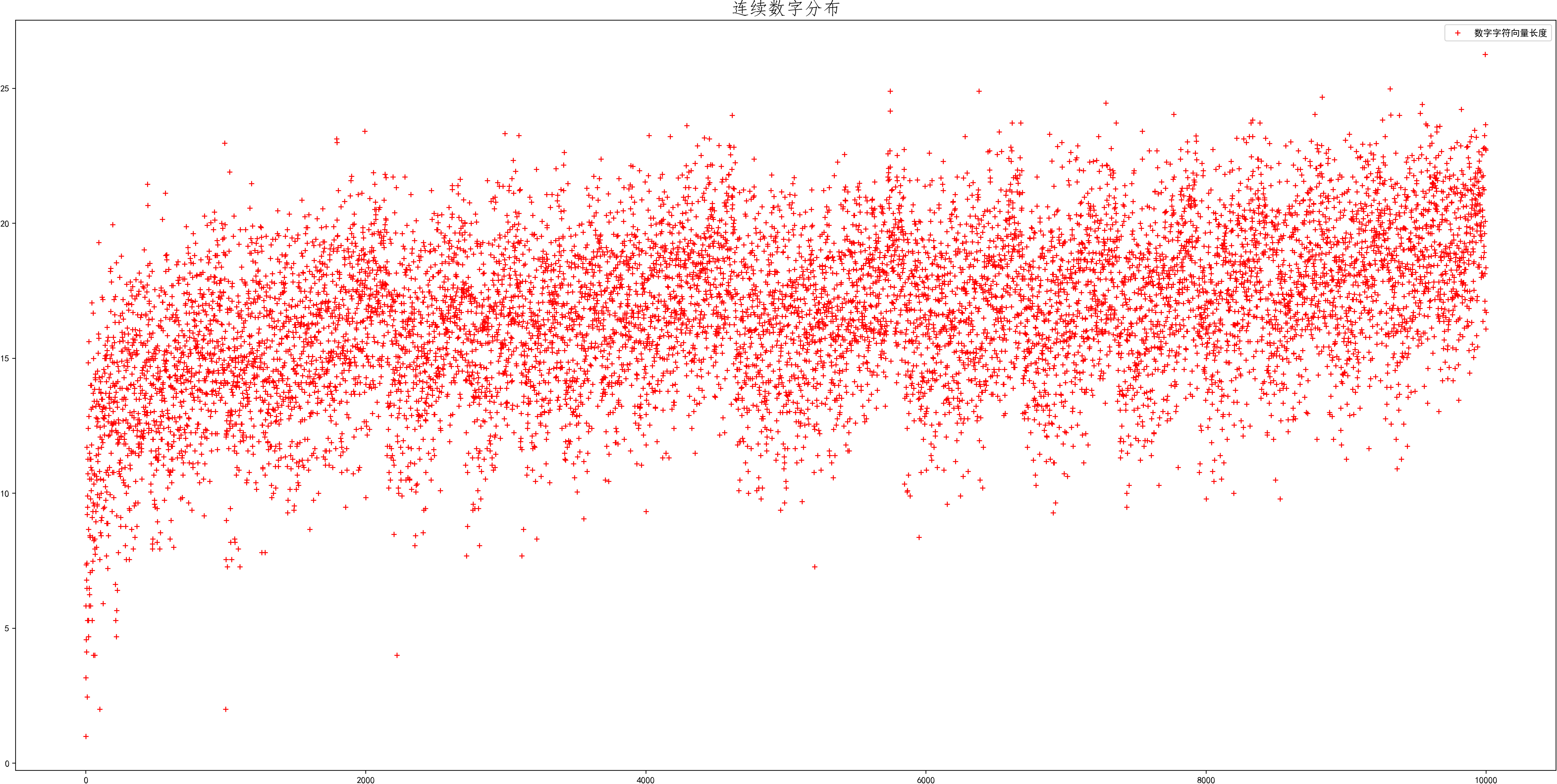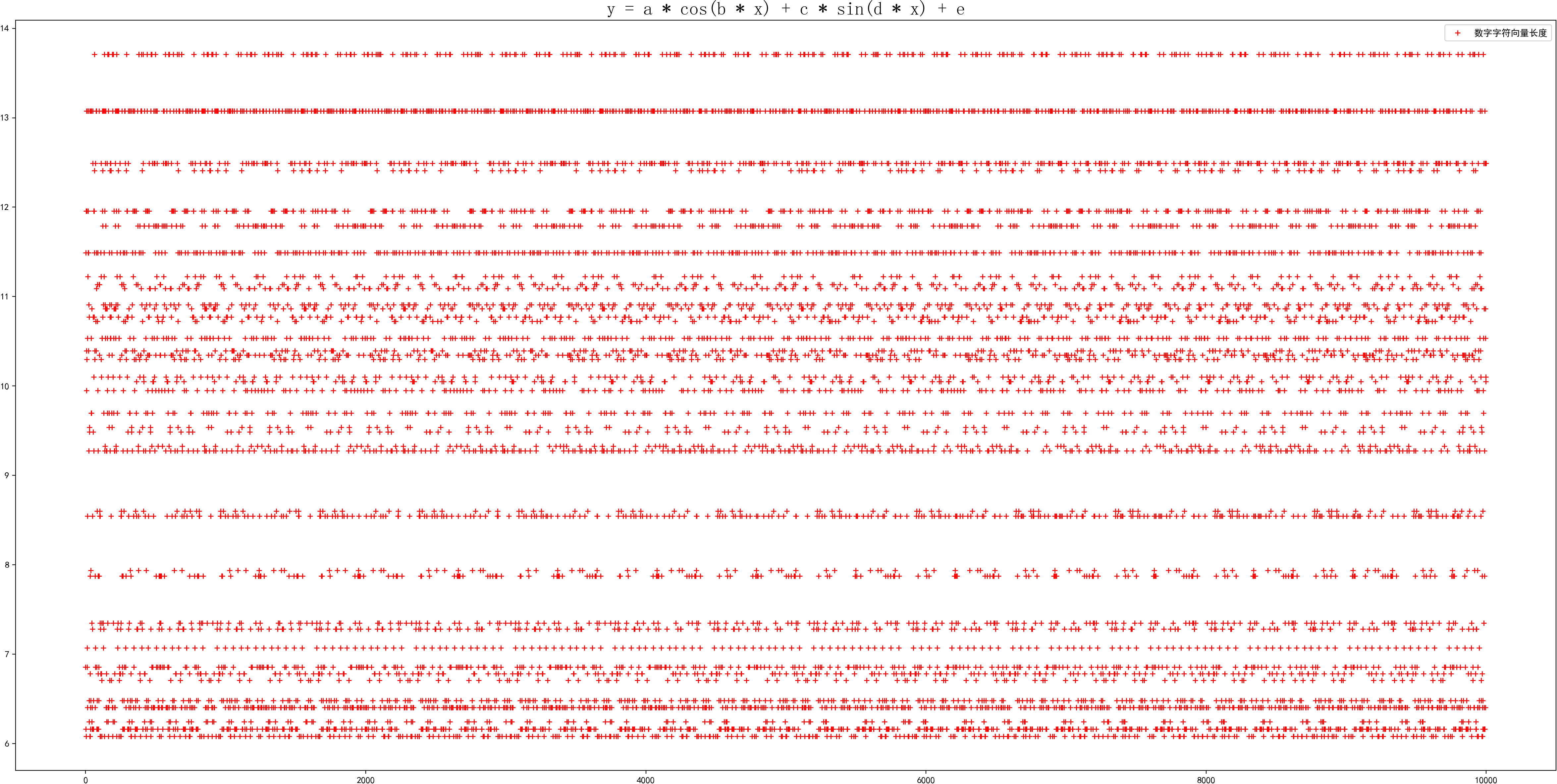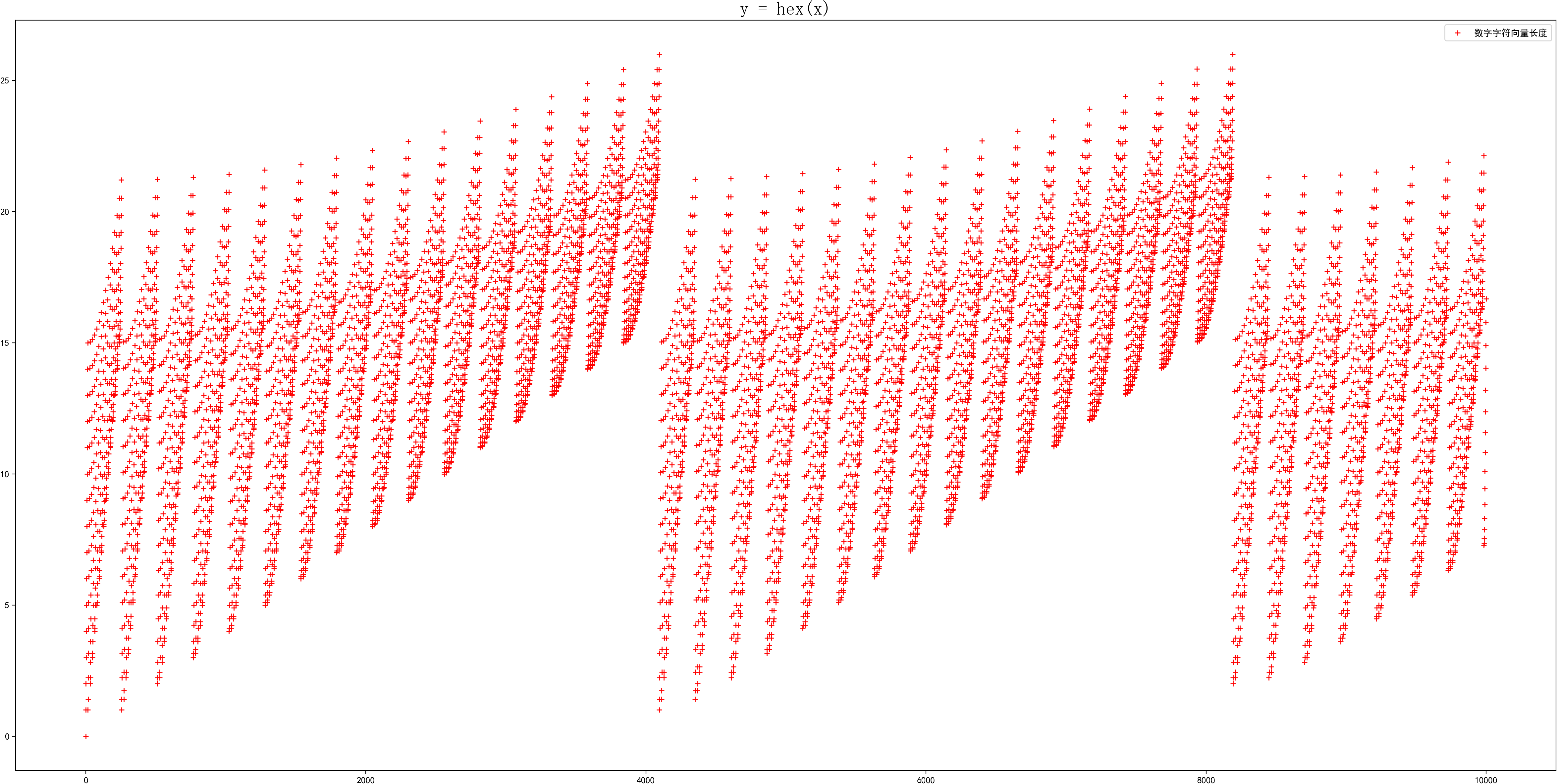---分割线---